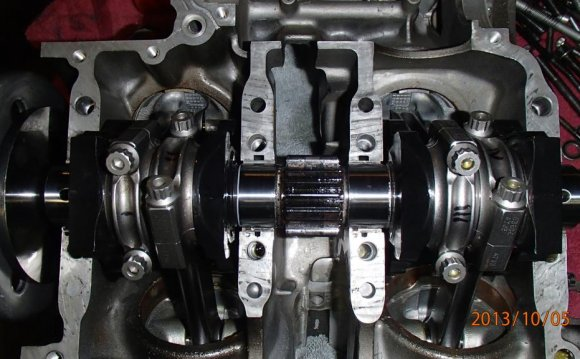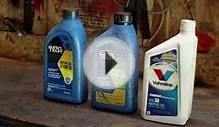# Types of IC Engines

January 30, 2022With a growing demand for transportation IC engines have gained lot of importance in automobile industry. It is therefore necessary to produce efficient and economical engines. While developing an IC engine it is required to take in consideration all the parameters affecting the engines design and performance. There are enormous parameters so it becomes difficult to account them while designing an engine. So it becomes necessary to conduct tests on the engine and determine the measures to be taken to improve the engines performance.

### Performance parameters

1. Power and Mechanical Efficiency

2. Fuel Air Ratio

3. Volumetric Efficiency

4. Specific Output

5. Specific Fuel Consumption

6. Thermal Efficiency and Heat Balance

7. Exhaust Smoke and Emissions

8. Effective Pressure and Torque

### Power and mechanical efficiency

An IC engine is used to produce mechanical power by combustion of fuel. Power is referred to as the rate at which work is done. Power is expressed as the product of force and linear velocity or product of torque and angular velocity. In order to measure power one needs to measure torque or force and speed. The force or torque is measured by Dynamometer and speed by Tachometer. The power developed by an engine and measured at the output shaft is called the brake power (bp) and is given by,

where:

is the torque, in Newton meter (N.m), is the rotational speed, in minutes, is the brake power, in watt.

However while calculating the Mechanical efficiency another factor called Indicated Power (ip) is considered. It is defined as the power developed by combustion of fuel in the cylinder of engine is called (ip). It is always more than brake power. It is given by,

is the mean pressure, is the area of the piston is the number of cylinders

Therefore, the difference between ip and bp indicates the power loss in the mechanical components of engine (due to friction). So the mechanical efficiency is defined as ratio of brake power to the indicated power.

or :

Friction power is the difference between indicated power and brake power.

### Air–fuel ratio

It is the ratio of mass of fuel to mass or volume of air in mixture. It affects the phenomenon of combustion and used for determining flame propagation velocity, the heat released in combustion chamber. For practise always relative air fuel ratio is defined. It is the ratio of actual air–fuel ratio to that of the stoichiometric air fuel ratio required for burning of fuel which is supplied.

Equivalence ratio, Ø :

Relative ratio, λ :

### Thermal efficiency and heat balance

It is the ratio of output to that of energy input in the form of fuel. It gives the efficiency with which the chemical energy of fuel is converted into mechanical work. It shows that all chemical energy of fuel is not converted into heat energy.

Thermal efficiency and total energy input- The methodology for calculating thermal efficiency of a unit is described in this section to help to determine whether the unit qualifies to exemption or not. It also includes total energy input which also helps in determining thermal efficiency.

The thermal efficiency standard for a unit are decided by CAIR model trading rules, the CAIR FIP, CAMR, the CAMR Hg model trading rule, and the proposed CAMR Federal Plan, EPA. However, the thermal efficiency standard applicable to all fuels combusted by a unit are decided by the United States Environmental Protection Agency (EPA), while the application of the standard to natural gas and oil are made by FERC.

It is defined as the amount of fuel consumed for each unit of brake power per hour; it indicates the efficiency with which the engine develops the power from fuel. It is used to compare performance of different engines.

The amount of fuel which an engine consumes is rated by its brake specific fuel consumption (BSFC). In the U.S. this is generally computed in dimensions of pounds of fuel per horsepower per hour. For most internal combustion engines the BSFC will be in the range of 0.5 to 0.6. This estimate fits well with the old rule of thumb that an engine will burn HP/10 gallons per hour. Here is an example with a 90 HP engine. When developing 90 HP, how much fuel will it burn? Assume a BSFC of 0.55 and gasoline at 6.25 lbs/gallon:

• 90 x 0.55 = 49.5 pounds of fuel burned per hour
• 49.5 x 1/6.25 = 7.92 gallons per hour
Source: en.wikipedia.org
##### RELATED VIDEOWhat types of oil to use in small engines (4 stroke motor ...Petter M-Type Engine - StationärmotorCrankshaft of V12 Engine